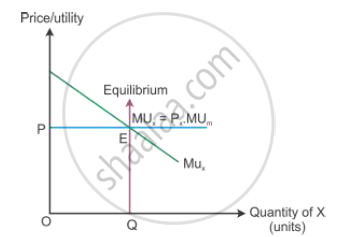Share

# Explain the Condition of Consumer'S Equilibrium with the Help of Utility Analysis. - CBSE (Arts) Class 12 - Economics

ConceptConditions of Consumer's Equilibrium Using Marginal Utility Analysis

#### Question

Explain the condition of consumer's equilibrium with the help of utility analysis.

#### Solution

Given the price of the good, a consumer will decide the amount of goods to buy. So, the consumer compares the price of the good with its utility. A rational consumer will be at equilibrium only when the marginal utility is equal to the price paid for the good.

MUX = PX

The marginal utility is greater than the price paid for the good, i.e. MUX > PX implies that the consumer is not in equilibrium and buys more of a good. While the marginal utility is lesser than the price paid for the good, i.e. MUX < PX implies that the consumer is not in equilibrium and buys less of that good.In the diagram, OP is the price of the good given on the Y-axis and OQ is the utility given on the X-axis. The marginal utility curve MUX slopes downwards because the marginal utility diminishes with every additional consumption of X. The consumer reaches equilibrium at Point E, where the marginal utility is equal to the price paid for the good.

Is there an error in this question or solution?

#### APPEARS IN

Solution Explain the Condition of Consumer'S Equilibrium with the Help of Utility Analysis. Concept: Conditions of Consumer's Equilibrium Using Marginal Utility Analysis.
S